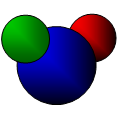CGAL 4.12 - 3D Envelopes
3D Envelopes ReferenceDan Halperin, Michal Meyerovitch, Ron Wein, and Baruch Zukerman
This package consits of functions that compute the lower (or upper) envelope of a set of arbitrary surfaces in 3D. The output is represented as an 2D envelope diagram, namely a planar subdivision such that the identity of the surfaces that induce the envelope over each diagram cell is unique.

Introduced in: CGAL 3.3
Depends on: 2D Arrangements
BibTeX: cgal:mwz-e3-18a
Windows Demos: 3D Envelopes, L1 Voronoi Diagram
Common Demo Dlls: dlls

This package consists of functions that compute the lower (or upper) envelope of a set of arbitrary surfaces in 3D. The output is represented as an 2D envelope diagram - a planar subdivision such that the identity of the surfaces that induce the envelope over each diagram cell is unique.

## Functions

• CGAL::lower_envelope_3
• CGAL::upper_envelope_3
• CGAL::lower_envelope_xy_monotone_3
• CGAL::upper_envelope_xy_monotone_3

## Concepts

• EnvelopeTraits_3

## Classes

• CGAL::Envelope_diagram_2<EnvTraits>
• CGAL::Env_triangle_traits_3<Kernel>
• CGAL::Env_sphere_traits_3<ConicTraits>
• CGAL::Env_plane_traits_3<Kernel>
• CGAL::Env_surface_data_traits_3<Traits,XyData,SData,Cnv>

Concepts

## Classes

class  CGAL::Env_plane_traits_3< Kernel >
The traits class Env_plane_traits_3 models the EnvelopeTraits_3 concept, and is used for the construction of lower and upper envelopes of planes and half planes in the space. More...

class  CGAL::Env_sphere_traits_3< ConicTraits >
The traits class Env_sphere_traits_3 models the EnvelopeTraits_3 concept, and is used for the construction of lower and upper envelopes of spheres. More...

class  CGAL::Env_surface_data_traits_3< Traits, XyData, SData, Cnv >
The class Env_surface_data_traits_3 is a model of the EnvelopeTraits_3 concept and serves as a decorator class that allows the extension of the surfaces defined by the base traits-class (the Traits parameter), which serves as a geometric traits-class (a model of the EnvelopeTraits_3 concept), with extraneous (non-geometric) data fields. More...

class  CGAL::Env_triangle_traits_3< Kernel >
The traits class Env_triangle_traits_3 models the EnvelopeTraits_3 concept, and is used for the construction of lower and upper envelopes of triangles in the space. More...

class  CGAL::Envelope_diagram_2< EnvTraits >
The class-template Envelope_diagram_2 represents the minimization diagram that corresponds to the lower envelope of a set of curves, or the maximization diagram that corresponds to their upper envelope. More...

## Functions

template<class InputIterator , class Traits >
void CGAL::lower_envelope_3 (InputIterator begin, InputIterator end, Envelope_diagram_2< Traits > &diag)
Computes the lower envelope of a set of surfaces in $$\mathbb{R}^3$$, as given by the range [begin, end). More...

template<class InputIterator , class Traits >
void CGAL::lower_envelope_xy_monotone_3 (InputIterator begin, InputIterator end, Envelope_diagram_2< Traits > &diag)
Computes the lower envelope of a set of $$xy$$-monotone surfaces in $$\mathbb{R}^3$$, as given by the range [begin, end). More...

template<class InputIterator , class Traits >
void CGAL::upper_envelope_3 (InputIterator begin, InputIterator end, Envelope_diagram_2< Traits > &diag)
Computes the upper envelope of a set of surfaces in $$\mathbb{R}^3$$, as given by the range [begin, end). More...

template<class InputIterator , class Traits >
void CGAL::upper_envelope_xy_monotone_3 (InputIterator begin, InputIterator end, Envelope_diagram_2< Traits > &diag)
Computes the upper envelope of a set of $$xy$$-monotone surfaces in $$\mathbb{R}^3$$, as given by the range [begin, end). More...

## ◆ lower_envelope_3()

template<class InputIterator , class Traits >
 void CGAL::lower_envelope_3 ( InputIterator begin, InputIterator end, Envelope_diagram_2< Traits > & diag )

#include <CGAL/envelope_3.h>

Computes the lower envelope of a set of surfaces in $$\mathbb{R}^3$$, as given by the range [begin, end).

The lower envelope is represented using the output minimization diagram diag.

Template Parameters
 Traits must be a model of EnvelopeTraits_3. InputIterator must be an input iterator with value type Traits::Surface_3.
Examples:
Envelope_3/envelope_planes.cpp, Envelope_3/envelope_spheres.cpp, and Envelope_3/envelope_triangles.cpp.

## ◆ lower_envelope_xy_monotone_3()

template<class InputIterator , class Traits >
 void CGAL::lower_envelope_xy_monotone_3 ( InputIterator begin, InputIterator end, Envelope_diagram_2< Traits > & diag )

#include <CGAL/envelope_3.h>

Computes the lower envelope of a set of $$xy$$-monotone surfaces in $$\mathbb{R}^3$$, as given by the range [begin, end).

The lower envelope is represented using the output minimization diagram diag.

Template Parameters
 Traits must be a model of EnvelopeTraits_3. InputIterator must be an input iterator with value type Traits::Xy_monotone_surface_3.

## ◆ upper_envelope_3()

template<class InputIterator , class Traits >
 void CGAL::upper_envelope_3 ( InputIterator begin, InputIterator end, Envelope_diagram_2< Traits > & diag )

#include <CGAL/envelope_3.h>

Computes the upper envelope of a set of surfaces in $$\mathbb{R}^3$$, as given by the range [begin, end).

The upper envelope is represented using the output maximization diagram diag.

Template Parameters
 Traits must be a model of EnvelopeTraits_3. InputIterator must be an input iterator with value type Traits::Surface_3.
Examples:
Envelope_3/envelope_planes.cpp, and Envelope_3/envelope_triangles.cpp.

## ◆ upper_envelope_xy_monotone_3()

template<class InputIterator , class Traits >
 void CGAL::upper_envelope_xy_monotone_3 ( InputIterator begin, InputIterator end, Envelope_diagram_2< Traits > & diag )

#include <CGAL/envelope_3.h>

Computes the upper envelope of a set of $$xy$$-monotone surfaces in $$\mathbb{R}^3$$, as given by the range [begin, end).

The lower envelope is represented using the output maximization diagram diag.

Template Parameters
 Traits must be a model of EnvelopeTraits_3. InputIterator must be an input iterator with value type Traits::Xy_monotone_surface_3.